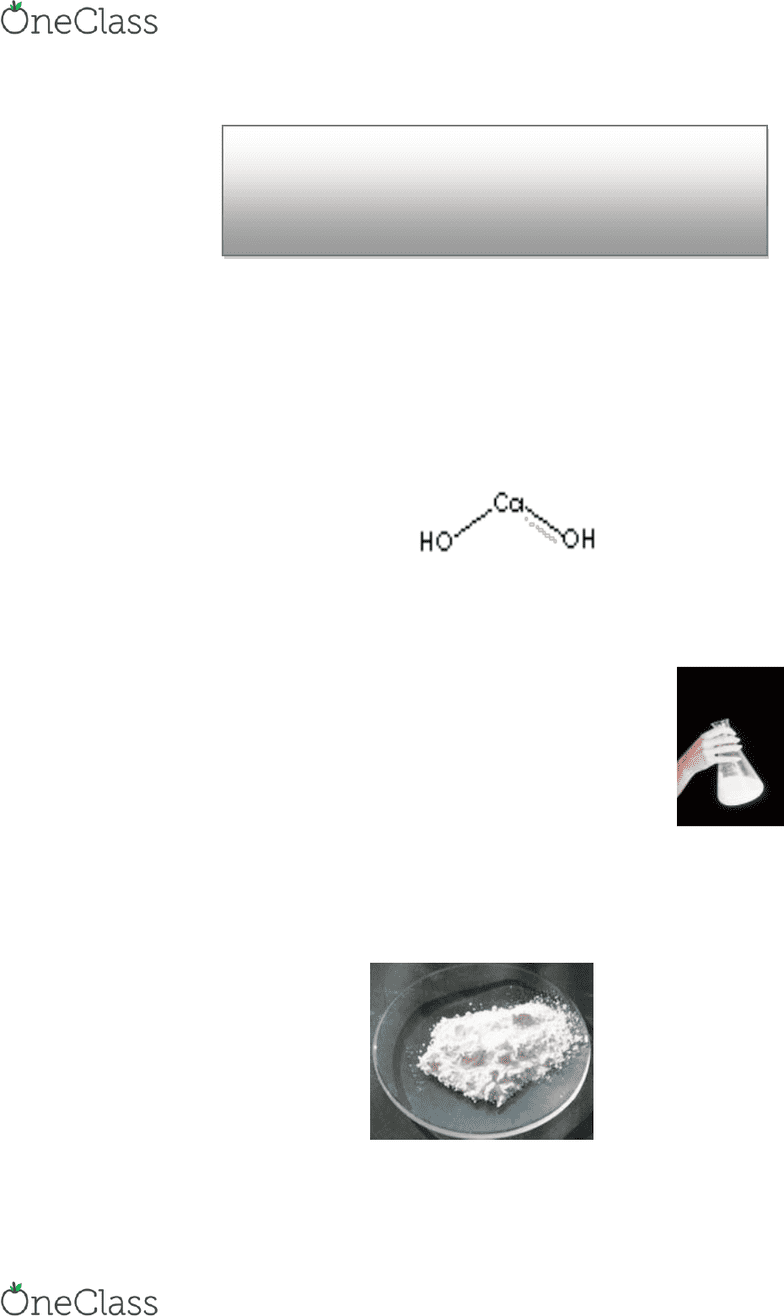Class Notes (1,100,000)
CA (620,000)
UTM (20,000)
Chemistry (700)
CHM110H5 (200)
Lecture 2

# CHM110H5 Lecture Notes - Lecture 2: Distilled Water, Titration, Equivalence Point

Department
Chemistry
Course Code
CHM110H5
Professor
Lecture
2

This preview shows pages 1-2. to view the full 7 pages of the document.Introduction:
Calcium hydroxide is a soft white caustic powder used in making mortar, cements, calcium salts,
paints, and petrochemicals. It is also used in saltwater aquaria to make up kalkwasser/limewater
solutions for reef tanks, and is used as a pH regulating agent. Notice that calcium hydroxide is
divalent and thus has twice the neutralizing power as molecules like NaOH that are monovalent.
A Calcium Hydroxide Molecule:
Calcium hydroxide is manufactured industrially by adding water to calcium oxide (quicklime) in a
strongly exothermic reaction:
CaO(s) + H2O(l) Ca(OH)2(s)
Calcium hydroxide, Ca(OH)2, is an ionic solid that is only slightly soluble in water.
Solid Calcium Hydroxide:
The Ksp of Calcium Hydroxide by Titration of
HCl with saturated Ca(OH)2
C12-4-13
find more resources at oneclass.com
find more resources at oneclass.com

Only pages 1-2 are available for preview. Some parts have been intentionally blurred.A calcium hydroxide solution is also referred to as limewater. A saturated solution of calcium
hydroxide has the solid in equilibrium with its ions as shown below:
Ca(OH)2(s) Ca2+(aq) + 2OH-(aq)
Recall that a saturated solution is a solution that contains the maximum amount of dissolved
solute possible at a given temperature. (The solution contains undissolved solute in equilibrium
with the solution.)
Since calcium hydroxide is only slightly soluble in water, it is a difficult base to classify. It is often
assumed that since calcium hydroxide has a low solubility that it is a weak base. But, don’t forget
that it contains hydroxides ions, which automatically makes it a strong base! In fact, the pH of a
saturated calcium hydroxide solution is about 12.4. Thus we can classify a saturated solution of
calcium hydroxide as a dilute solution of a strong base.
A molecular view of a saturated solution of calcium hydroxide would look similar to the following,
with clumps of undissolved calcium hydroxide at the bottom of the beaker in equilibrium with
dissolved Ca2+ and OH- ions (although their would be twice as many OH- ions than Ca2+ ions):
Note that the rate of dissolving is equal to the rate of precipitation in a saturated solution
equilibrium.
In this type of equilibrium, the equilibrium constant is called the solubility product, and is
represented by the symbol Ksp. Whenever you see the symbol Ksp it refers to a solubility
equation, written with the solid to the left of the equilibrium sign, and the dissolved products to the
right. The Ksp for this reaction will be:
Ksp = [Ca2+][OH-]2
(The solid state is not included in a Ksp expression since it is a pure substance and cannot be
expressed as a concentration).
Note that substances that have a large Ksp value have a higher solubility (more dissolved ions);
and substances with a small Ksp value have a lower solubility (few dissolved ions).
Every substance that forms a saturated solution will have a Ksp. However, for very soluble
substances like NaCl, the value is so large that the concept is rarely used. In low solubility
substances, the value of Ksp is a useful quantity that lets us predict and calculate solubilities of
substances in solution. In this experiment you will collect the data that allows you to calculate the
solubility of Ca(OH)2, and its Ksp value.
find more resources at oneclass.com
find more resources at oneclass.com
###### You're Reading a Preview

Unlock to view full version